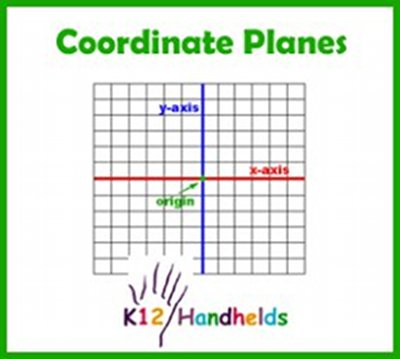www.k12handhelds.com
Phone: 800-679-2226

Copyright © 2006 by K12 Handhelds, Inc. License CC-by,Developed in conjunction with Monroe 2-Orleans BOCES.

# Coordinate Planes

Graphs are drawn on a grid called a coordinate plane. Grids, or coordinate planes, are also used for maps. The different squares on the grid are numbered so that they can be used to identify where different points are.
There are two main number lines on a coordinate plane. The one that runs from right to left or horizontally is called the x-axis. The one that runs up and down or vertically is called the y-axis. The center point where they meet is the origin.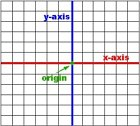The coordinate plane is divided up into four areas called quadrants. Each quadrant is given a number. Quadrant I is the upper-right area. Quadrant II is the upper-left area. Quadrant III is the lower-left area. Quadrant IV is the lower-right area. It is easiest to remember these by starting in the upper-right corner and moving counter-clockwise (the opposite of the way the hands on the clock go).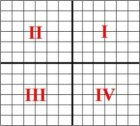We can mark different points on the grid. To tell the location of points, we use ordered pairs of numbers. An ordered pair looks like this:

(2, 3)

The first number tells the location on the x-axis. The second number tells the location on the y-axis. Use the number to count how many points on the grid to move.

Here is how we plot (2, 3):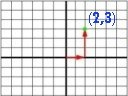If the first number is negative, we move to the left on the x-axis. Here is the plot of (-4, 3):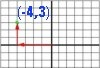If the second number in the pair is negative, we move down on the y-axis. Here is the plot of (3, -5):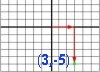Sometimes, coordinate planes have different scales, so that each line stands for more than one unit. For example, in this grid, each line is 10 units: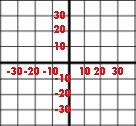Now let’s practice.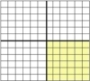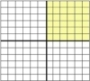Give the ordered pair for the point on this grid.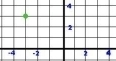Give the ordered pair for the point on this grid.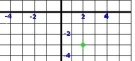Give the ordered pair for the point on this grid.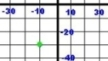This is the end of the Coordinate Planes ebook.